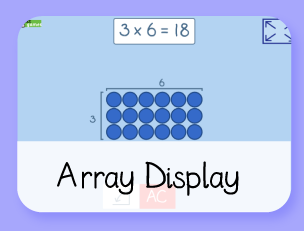# Doggy Division Dinners

### A simple introduction to division in real-life situations

Learning Objectives:
• Y1: To solve one-step problems involving multiplication and division, by calculating the answer using concrete objects, pictorial representations and arrays with the support of the teacher.
• Y2: To recall and use multiplication and division facts for the 2, 5 and 10 multiplication tables, including recognising odd and even numbers
• Y2: To solve problems involving multiplication and division, using materials, arrays, repeated addition, mental methods, and multiplication and division facts, including problems in contexts.
A simple introduction to division, (through sharing). The dogs are always hungry, there is no end to this game!

There are two types of division, 'Grouping' and 'Sharing'. Sharing is often used as a first introduction to division in real life situations. This game focusses on 'Sharing', e.g. 10 dog biscuits are shared between 2 dogs. How many biscuits do they get each?

NB: If I were to solve 10 ÷ 2 via the 'Grouping' technique it would be... 'There are 10 dog biscuits. Dogs are allowed 5 biscuits each. How many dogs could you feed?'

### Instructions:

Read the Maths Sentence on the box of dog food, then share out the bones between all of the dogs.

NB: In Year 2 children are expected to use their knowledge of multiplication facts for 2, 5 and 10. I could have focussed on having only 2, 5 or 10 dogs, but I wanted this to be more of an introduction to division rather than a place to use inverse multiplication facts. More able children in Year 2 will find this too simple.

Have fun!

Build on the learning with
these games:Made using theandlibraries.